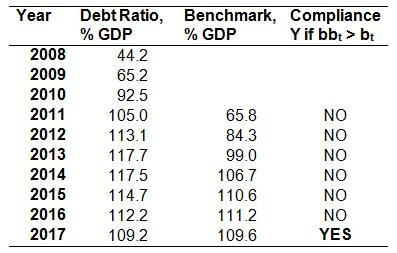## Friday, May 4, 2012

### Complying with the Debt Reduction Rule

The issue of whether the Fiscal Compact will mean additional austerity in the post-2015 period has generated some heat in the referendum debate.  John has usefully provided some light to this issue in a previous post.  This post adds little to the conclusions there on the “1/20th” rule but relays a similar point in a slightly different way.  Based on IMF projections Ireland will satisfy the debt reduction rule in 2015.

The debt reduction benchmark is calculated as an average over a three-year period.  One of two averages can be used to satisfy the rule.  There is a backward-looking average covering the years t-1, t-2 and t-3 with a benchmark calculated for year t, and there is also a partially-forward-looking average for the years t-1, t and t+1 with a benchmark calculated for year t+2.

The formula for the benchmark is in the Code of Conduct for the Stability and Growth Pact and for the retrospective average it can be seen on page 8 to be:where bb is the benchmark or target debt ratio and b is the debt-to-GDP ratio in other years.  Although there is a bit to the formula all that is needed is the debt ratios for three years in order to calculate the benchmark for the next year.

If the debt ratio for the current year is expected to be below the benchmark level given by the formula then the conditions of the debt reduction rule are satisfied.

To simulate the impact of the rule on Ireland we can use the IMF’s forecasts of the general government gross debt from the recent update of the World Economic Outlook as these extend out to 2017.  We will use these to gauge Ireland’s performance to the rule beginning in 2012.The debt ratio column are actual data up to 2010 and are the IMF’s projections from 2011 to 2017.  The benchmark column are the targets for each year and is calculated by putting the debt ratios for the preceding three years into the formula shown above.   Compliance is true if the debt ratio for any year is less than the benchmark calculated for that year.  Under current assumptions and IMF projections Ireland will satisfy the retrospective version of the debt reduction rule in 2017.

One of the assumptions the IMF makes is that we undertake the €8.6 billion of fiscal adjustment planned for 2013-15.  Projections after that are based on a “no policy change” scenario.  Under IMF projections we will satisfy the debt brake rule in 2017 with no additional fiscal effort above what has already been provided for up to 2015 with neutral budgets after that.

The debt reduction rule can be satisfied while running deficits and does not require any debt repayments.  The IMF project that there will be an overall budget deficit of 1.9% of GDP in 2017.

The gross debt continues to rise and in the years from 2014 to 2017 (the years used in the 2017 comparison) the gross debt increases from €201.0 billion in 2014 to €213.5 billion in 2017.

If the alternative forward-looking version of the rule was applied it would actually show that we would be in compliance with the rule from 2015, as the benchmark calculation is based on the debt ratios in the same three years, 2014, 2015 and 2016 and again compared to the ratio in 2017.  Using the forward looking version of the rule in 2015 will also give a benchmark of 109.6% of GDP for 2017 which is, of course, above the projected debt ratio for 2017.

Although this is only a simulation it does show that we would not need additional fiscal adjustment to satisfy the debt brake rule.  In fact, using IMF projections it can be shown that we will be able to satisfy the rule before we even leave the Excessive Deficit Procedure (EDP).  The debt brake rule doesn’t actually become effective until three years after a country leaves the EDP.  We have until 2018 to become compliant with the debt reduction rule but we may actually be compliant by as early as 2015.

One reason for this is that the “1/20th” rule is actually relatively benign and according to Karl Whelan in section 2.1 of this paper the “rate of progress that is deemed satisfactory is still very slow.”  We have plenty to be worrying about but satisfying the conditions of the debt brake is not one of them.  In fact, it is likely that we will want to reduce the debt ratio at a rate faster than that required by the rule.